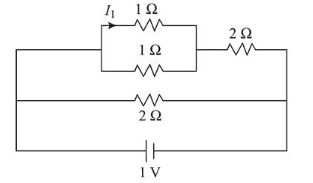# The current

Question:

The current $I_{1}$ (in $A$ ) flowing through $1 \Omega$ resistor in the following circuit is:1. (1) $0.4$

2. (2) $0.5$

3. (3) $0.2$

4. (4) $0.25$

Correct Option: , 3

Solution:

(3)